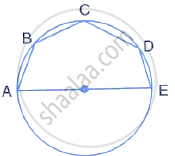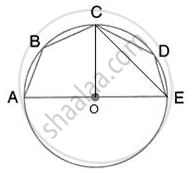Share

# In the Given Figure, Ae is the Diameter of the Circle. Write Down the Numerical Value of ∠Abc+∠Cde. Give Reasons for Your Answer. - Mathematics

Course

#### Question

In the given figure, AE is the diameter of the circle. Write down the numerical value of ∠ABC+∠CDE. Give reasons for your answer.#### SolutionJoin centre O and C and EC.
∠AOC = (180°)/2 = 90°
And ∠AOC = 2∠AEC
(Angle at the centre is double the angle at the circumference subtended by the same chord)

⇒∠AEC = (90°)/2 = 45°
Now, ABCE is a cyclic quadrilateral
∴ ∠ABC + ∠AEC = 180°
(pair of opposite angles in a cyclic quadrilateral are supplementary)
⇒ ∠ABC =180° - 45° =135°
Similarly, ∠CDE = 135°
∴ ∠ABC + CDE -135° +135° = 270°

Is there an error in this question or solution?